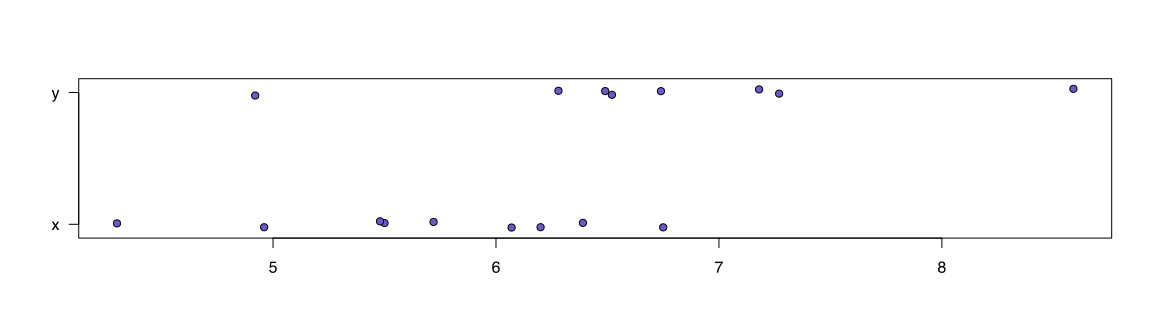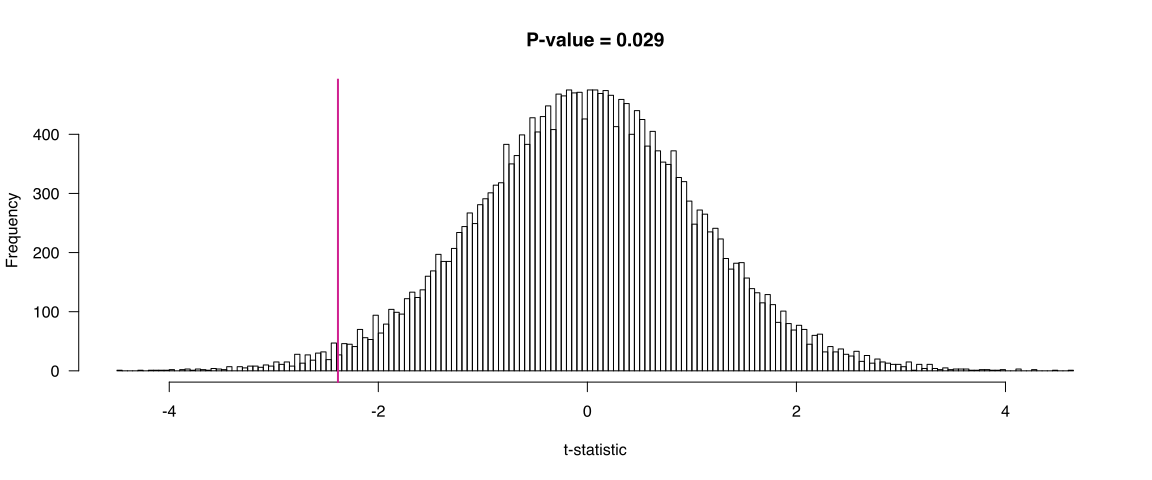Problem 3 in this week’s homework said, “Take the script from the last problem in homework 2 and turn it into an R Markdown document.” Here’s my solution.

The script performed an exhaustive permutation test (with the t-statistic) and plotted the results.

I’m going to use default chunk options of fig.width=12 (wider figure width) and dev="svg" (use SVG for the figures). Normally I’d use include=FALSE to hide this code chunk, but here I’ll leave it in the output.

knitr::opts_chunk\$set(fig.width=12, dev="svg")

Two utility functions were defined, binary.v() and perm.test(). I’ll define them here, but in a way that will be hidden in the output (using the chunk option echo=FALSE).

We first create the data objects

x <- c(6.20, 5.72, 6.07, 6.75, 5.50, 6.39, 4.30, 4.96, 5.48)
y <- c(6.49, 6.52, 6.28, 8.59, 7.18, 4.92, 6.74, 7.27)

Here’s a plot of the data, using stripchart(). I use set.seed() so that it will appear exactly the same way every time. (The permutation results below will also then be the same every time I compile this document.)

set.seed(99693682)
stripchart(list(x=x, y=y), method="jitter", jitter=0.03,
pch=21, bg="slateblue", las=1)We call perm.test() to run the permutation test.

permt <- perm.test(x, y)

We grab the observed t-statistic (which was saved as attribute)

tobs <- attr(permt, "tobs")

We can calculate the p-value from the permutation test, as the proportion of t-statistics from the permutations that were greater or equal to the observed t-statistic, in absolute value. Note that the nominal p-value is 0.036

pval <- mean(abs(permt) >= abs(tobs))

The observed t-statistic was -2.39. The p-value, for the test of whether the two population averages were different, was 0.029.

I’ll save the results to a file, but I’ll hide this in the output.

I’ll plot the permutation results, with a vertical line at the observed t-statistic.

hist(permt, breaks=200, xlab="t-statistic", las=1,
main = paste("P-value =", round(pval, 3)))
abline(v=tobs, lwd=2, col="violetred")### Session info

I try to remember to end every R Markdown document with information about the R and package versions that were used. R is distributed with the sessionInfo() function (in the utils package); I prefer devtools::session_info(), as the output is nicer, but I won’t use it here.

sessionInfo()
## R version 3.1.2 Patched (2014-12-11 r67157)
## Platform: x86_64-apple-darwin13.4.0 (64-bit)
##
## locale:
##  C
##
## attached base packages:
##  stats     graphics  grDevices utils     datasets  methods   base
##
## loaded via a namespace (and not attached):
##  digest_0.6.8    evaluate_0.5.5  formatR_1.0     htmltools_0.2.6
##  knitr_1.9       rmarkdown_0.5.1 stringr_0.6.2   tools_3.1.2
##  yaml_2.1.13